Anzeige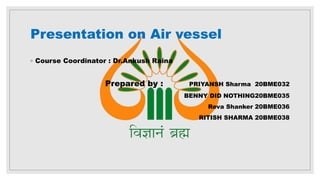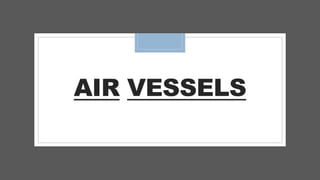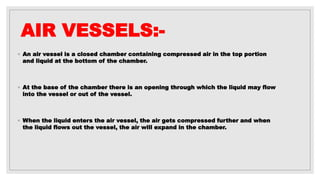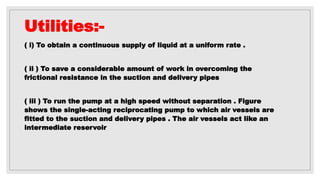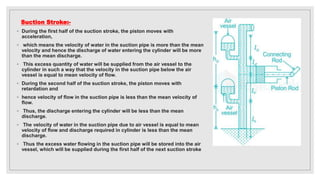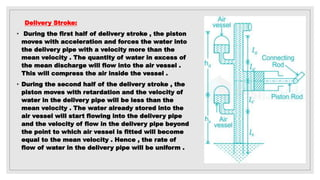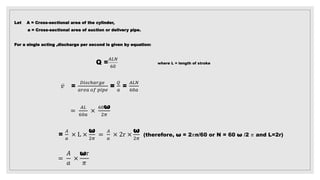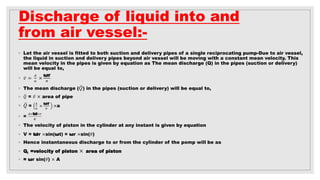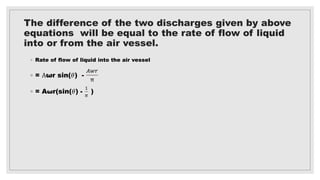1 von 10
Anzeige

### air vessel and into and out flow in air vessel.pptx

1. Presentation on Air vessel ◦ Course Coordinator : Dr.Ankush Raina Prepared by : PRIYANSH Sharma 20BME032 BENNY DID NOTHING20BME035 Reva Shanker 20BME036 RITISH SHARMA 20BME038
2. AIR VESSELS
3. AIR VESSELS:- ◦ An air vessel is a closed chamber containing compressed air in the top portion and liquid at the bottom of the chamber. ◦ At the base of the chamber there is an opening through which the liquid may flow into the vessel or out of the vessel. ◦ When the liquid enters the air vessel, the air gets compressed further and when the liquid flows out the vessel, the air will expand in the chamber.
4. Utilities:- ( i) To obtain a continuous supply of liquid at a uniform rate . ( ii ) To save a considerable amount of work in overcoming the frictional resistance in the suction and delivery pipes ( iii ) To run the pump at a high speed without separation . Figure shows the single-acting reciprocating pump to which air vessels are fitted to the suction and delivery pipes . The air vessels act like an intermediate reservoir
5. Suction Stroke:- ◦ During the first half of the suction stroke, the piston moves with acceleration, ◦ which means the velocity of water in the suction pipe is more than the mean velocity and hence the discharge of water entering the cylinder will be more than the mean discharge. ◦ This excess quantity of water will be supplied from the air vessel to the cylinder in such a way that the velocity in the suction pipe below the air vessel is equal to mean velocity of flow. ◦ During the second half of the suction stroke, the piston moves with retardation and ◦ hence velocity of flow in the suction pipe is less than the mean velocity of flow. ◦ Thus, the discharge entering the cylinder will be less than the mean discharge. ◦ The velocity of water in the suction pipe due to air vessel is equal to mean velocity of flow and discharge required in cylinder is less than the mean discharge. ◦ Thus the excess water flowing in the suction pipe will be stored into the air vessel, which will be supplied during the first half of the next suction stroke
6. Delivery Stroke: • During the first half of delivery stroke , the piston moves with acceleration and forces the water into the delivery pipe with a velocity more than the mean velocity . The quantity of water in excess of the mean discharge will flow into the air vessel . This will compress the air inside the vessel . • During the second half of the delivery stroke , the piston moves with retardation and the velocity of water in the delivery pipe will be less than the mean velocity . The water already stored into the air vessel will start flowing into the delivery pipe and the velocity of flow in the delivery pipe beyond the point to which air vessel is fitted will become equal to the mean velocity . Hence , the rate of flow of water in the delivery pipe will be uniform .
7. Let A = Cross-sectional area of the cylinder, a = Cross-sectional area of suction or delivery pipe. For a single acting ,discharge per second is given by equation: Q = 𝐴𝐿𝑁 60 where L = length of stroke 𝑣 = 𝐷𝑖𝑠𝑐ℎ𝑎𝑟𝑔𝑒 𝑎𝑟𝑒𝑎 𝑜𝑓 𝑝𝑖𝑝𝑒 = 𝑄 𝑎 = 𝐴𝐿𝑁 60𝑎 = 𝐴𝐿 60𝑎 × 60ω 2𝜋 = 𝐴 𝑎 × L × ω 2𝜋 = 𝐴 𝑎 × 2r × ω 2𝜋 (therefore, ω = 2𝜋n/60 or N = 60 ω /2 𝜋 and L=2r) = 𝐴 𝑎 × ωr 𝜋
8. Discharge of liquid into and from air vessel:- ◦ Let the air vessel is fitted to both suction and delivery pipes of a single reciprocating pump-Due to air vessel, the liquid in suction and delivery pipes beyond air vessel will be moving with a constant mean velocity. This mean velocity in the pipes is given by equation as The mean discharge (Q) in the pipes (suction or delivery) will be equal to, ◦ 𝑣 = 𝐴 𝑎 × ωr 𝜋 ◦ The mean discharge (𝑄) in the pipes (suction or delivery) will be equal to, ◦ 𝑄 = 𝑣 × area of pipe ◦ 𝑄 = 𝐴 𝑎 × ωr 𝜋 ×a ◦ = 𝐴×ω×𝑟 𝜋 ◦ The velocity of piston in the cylinder at any instant is given by equation ◦ V = ωr ×sin(ωt) = ωr ×sin(𝜃) ◦ Hence instantaneous discharge to or from the cylinder of the pomp will be as ◦ Qi =velocity of piston × area of piston ◦ = ωr sin(𝜃) × A
9. The difference of the two discharges given by above equations will be equal to the rate of flow of liquid into or from the air vessel. ◦ Rate of flow of liquid into the air vessel ◦ = Aωr sin(𝜃) - 𝐴𝑤𝑟 π ◦ = Aωr(sin(𝜃) - 1 𝜋 )
10. THANK YOU
Anzeige